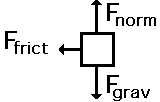Newton's Laws - Lesson 2 - Force and Its Representation

Drawing Free-Body Diagrams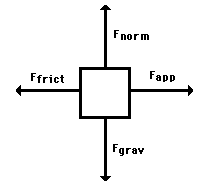Free-body diagrams are diagrams used to show the relative magnitude and direction of all forces acting upon an object in a given situation. A free-body diagram is a special example of the vector diagrams that were discussed in an earlier unit. These diagrams will be used throughout our study of physics. The size of the arrow in a free-body diagram reflects the magnitude of the force. The direction of the arrow shows the direction that the force is acting. Each force arrow in the diagram is labeled to indicate the exact type of force. It is generally customary in a free-body diagram to represent the object by a box and to draw the force arrow from the center of the box outward in the direction that the force is acting. An example of a free-body diagram is shown at the right

T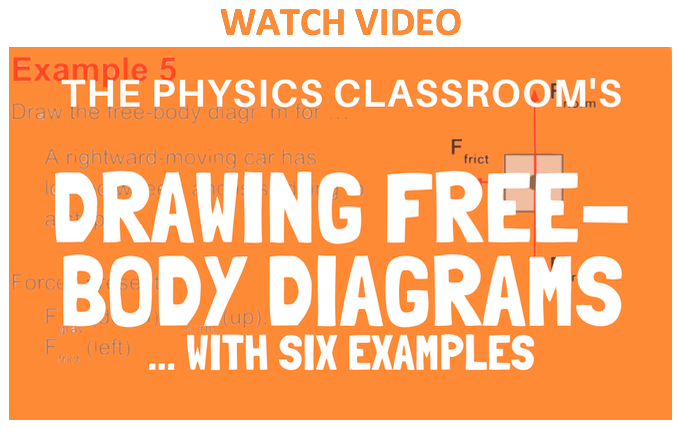he free-body diagram above depicts four forces acting upon the object. Objects do not necessarily always have four forces acting upon them. There will be cases in which the number of forces depicted by a free-body diagram will be one, two, or three. There is no hard and fast rule about the number of forces that must be drawn in a free-body diagram. The only rule for drawing free-body diagrams is to depict all the forces that exist for that object in the given situation. Thus, to construct free-body diagrams, it is extremely important to know the various types of forces. If given a description of a physical situation, begin by using your understanding of the force types to identify which forces are present. Then determine the direction in which each force is acting. Finally, draw a box and add arrows for each existing force in the appropriate direction; label each force arrow according to its type. If necessary, refer to the list of forces and their description in order to understand the various force types and their appropriate symbols.

We Would Like to Suggest ...Sometimes it isn't enough to just read about it. You have to interact with it! And that's exactly what you do when you use one of The Physics Classroom's Interactives. We would like to suggest that you combine the reading of this page with the use of our Free-Body Diagram Interactive. You can find it in the Physics Interactives section of our website. The Free-Body Diagram Interactive allows a learner to practice identifying the forces that act upon an object and to express such an understanding by the construction of a free-body diagram.

Practice

Apply the method described in the paragraph above to construct free-body diagrams for the various situations described below. Answers are shown and explained at the bottom of this page.

1. A book is at rest on a tabletop. Diagram the forces acting on the book. See answer.

2. A gymnast holding onto a bar, is suspended motionless in mid-air. The bar is supported by two ropes that attach to the ceiling. Diagram the forces acting on the combination of gymnast and bar. See answer.

3. An egg is free-falling from a nest in a tree. Neglect air resistance. Diagram the forces acting on the egg as it is falling. See answer.

4. A flying squirrel is gliding (no wing flaps) from a tree to the ground at constant velocity. Consider air resistance. Diagram the forces acting on the squirrel. See answer.

5. A rightward force is applied to a book in order to move it across a desk with a rightward acceleration. Consider frictional forces. Neglect air resistance. Diagram the forces acting on the book. See answer.

6. A rightward force is applied to a book in order to move it across a desk at constant velocity. Consider frictional forces. Neglect air resistance. Diagram the forces acting on the book. See answer.

7. A college student rests a backpack upon his shoulder. The pack is suspended motionless by one strap from one shoulder. Diagram the vertical forces acting on the backpack. See answer.

8. A skydiver is descending with a constant velocity. Consider air resistance. Diagram the forces acting upon the skydiver. See answer.

9. A force is applied to the right to drag a sled across loosely packed snow with a rightward acceleration. Neglect air resistance. Diagram the forces acting upon the sled. See answer.

10. A football is moving upwards towards its peak after having been booted by the punter. Neglect air resistance. Diagram the forces acting upon the football as it rises upward towards its peak. See answer.

11. A car is coasting to the right and slowing down. Neglect air resistance. Diagram the forces acting upon the car. See answer.

Answers to the above exercise are shown here. If you have difficulty drawing free-body diagrams, then you ought to be concerned. Continue to review the the list of forces and their description and this page in order to gain a comfort with constructing free-body diagrams.

1. A book is at rest on a tabletop. A free-body diagram for this situation looks like this: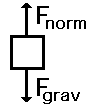2. A gymnast holding onto a bar, is suspended motionless in mid-air. The bar is supported by two ropes that attach to the ceiling. Diagram the forces acting on the combination of gymnast and bar. A free-body diagram for this situation looks like this: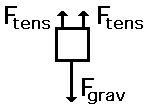3. An egg is free-falling from a nest in a tree. Neglect air resistance. A free-body diagram for this situation looks like this: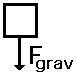4. A flying squirrel is gliding (no wing flaps) from a tree to the ground at constant velocity. Consider air resistance. A free-body diagram for this situation looks like this: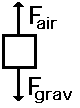5. A rightward force is applied to a book in order to move it across a desk with a rightward acceleration. Consider frictional forces. Neglect air resistance. A free-body diagram for this situation looks like this: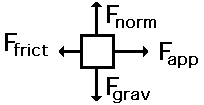6. A rightward force is applied to a book in order to move it across a desk at constant velocity. Consider frictional forces. Neglect air resistance. A free-body diagram for this situation looks like this: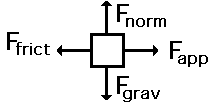7. A college student rests a backpack upon his shoulder. The pack is suspended motionless by one strap from one shoulder. A free-body diagram for this situation looks like this: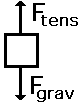8. A skydiver is descending with a constant velocity. Consider air resistance. A free-body diagram for this situation looks like this:9. A force is applied to the right to drag a sled across loosely packed snow with a rightward acceleration. Neglect air resistance. A free-body diagram for this situation looks like this:10. A football is moving upwards towards its peak after having been booted by the punter. Neglect air resistance. A free-body diagram for this situation looks like this:11. A car is coasting to the right and slowing down. Neglect air resistance. A free-body diagram for this situation looks like this: